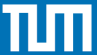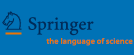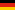Technische Universität MünchenSpringer-VerlagLeibniz Supercomputing Centre

# Colloquia on the Occasion of F. L. Bauer 85 Years - F. L. Bauer-Prize 2009 50 Years Numerische Mathematik

 Home Programme Registration Location

## Tuesday, June 16, 2009 Colloquium "50 Years Numerische Mathematik"

9:30 a.m. - 6 p.m., Leibniz Supercomputing Centre, Lecture Hall, Boltzmannstraße 1

Preliminary schedule:

• 9:30 Alfio Quarteroni
École Polytechnique Fédérale de Lausanne
Politecnico di Milano
Numerical Modeling through Domain Decomposition and Applications

Mathematical models of multiphysics problems can be conveniently accommodated in the framework of domain splitting. In this presentation I will introduce a general mathematical setting, discuss how domain decomposition algorithms and preconditioners can be called into play, then I will address some applications to the field of cardiovascular modeling and that of design and simulation for sports competition.

• 10:00 Douglas N. Arnold
University of Minnesota at Minneapolis
50 Years of Whitney Forms

Like Numerische Mathematik itself, the story of the Whitney forms (or Whitney elements) began about 50 years ago, has flourished since, and continues to be very active. In 1957 Hassler Whitney introduced these spaces of piecewise linear differential forms in order to study problems on the interface of algebraic topology and differential geometry. The Whitney forms have played a role in geometry ever since, including leading to the solution of an important conjecture. Independently, in 1975 Raviart and Thomas introduced their famous mixed finite elements, which were generalized to three dimensions in two separate ways by Nédélec in 1980, as the edge elements and the face elements. In 1988 Bossavit pointed out that these finite elements are exactly the Whitney forms. Whitney elements have proved to be hugely useful, and are particularly widely adopted by the practitioners in the computational electromagnetics community, who are now much greater users of them than the geometers. In this talk we will explain the origin and use of the Whitney forms in geometry, and describe how an understanding of their geometrical underpinnings is enabling major progress in numerical mathematics.

• 10:30 Marc A. Schweitzer
Universität Bonn
Meshfree Multilevel Methods for Partial Differential Equations

Meshfree methods have enjoyed a significant research effort in the past 20 years and substantial improvements have been accomplished. Many different numerical schemes have been proposed. For instance, the Diffuse Element Method, Smoothed Particle Hydrodynamics, Generalized Finite Difference Method, Radial Basis Functions, Reproducing Particle Kernel Method, Element Free Galerkin Method, Meshless Local Petrov Galerkin Method, Generalized/eXtended Finite Element Methods, or Partition of Unity Methods.

In this talk we review the two key concepts employed in the construction of the trial space of many meshfree Galerkin methods, the partition of unity approach and enrichment, which allow for the straightforward incorporation of non-polynomial shape functions in the approximation space. Thus, a priori information about singular or discontinuous behavior of the solution of a PDE can be encoded implicitly in the trial space and must not resolved by (adaptive) mesh refinement; i.e. we can employ an algebraic instead of geometric refinement of the PUM trial spaces which leads to a smaller number of unknowns. This is especially advantageous in a multilevel setting since singularities and discontinuities of the solution can be resolved easily on all levels. We apply the multilevel particle-partition of unity method to some reference problems from fracture mechanics to demonstrate the overall efficiency of this algebraic refinement approach.

• 11:00 Coffee break

• 11:30 Wolfgang Dahmen
RWTH Aachen
Compressed Sensing - Near Optimal Recovery of Signals from Highly Incomplete Measurements

The usual paradigm for signal processing is to model a signal as a bandlimited function and capture it signal by means of its time samples. The Shannon-Nyquist theory says that the sampling rate needs to be at least twice the bandwidth. For broadbanded signals, such high sampling rates may be impossible to implement in circuitry. Compressed Sensing is a new area of signal processing whose aim is to circumvent this dilemma by sampling signals closer to their information rate instead of their bandwidth. Rather than model the signal as bandlimited, Compressed Sensing assumes that the signal can be represented or approximated by a few suitably chosen terms from a basis expansion of the signal. It also enlarges the concept of sample to include the application of any linear functional applied to the signal.

We give a brief introduction to Compressed Sensing that centers on the effectiveness and implementation of random sampling. After briefly touching on the mathematical background, we discuss the notion of instance optimality as a performance benchmark that applies also to non-sparse signals. We sketch instance optimal decoding techniques with special emphasis on and thresholding techniques.

• 12:00 Carl W. R. de Boor
Issues in Multivariate Polynomial Interpolation

• 12:30 Lunch

• 14:10 G. W. (Pete) Stewart
University of Maryland
The Semidefinite B-Arnoldi Algorithm

• 14:40 Olof B. Widlund
New York University
Recent Advances on Domain Decomposition Algorithms for Almost Incompressible Elasticity

The domain decomposition methods considered are preconditioned conjugate gradient methods designed for the very large algebraic systems of equations which often arise in finite element practice. They are designed for massively parallel computer systems and the preconditioners are built from solvers on the substructures into which the domain of the given problem is partitioned. In addition, to obtain scalability, there must be a coarse problem, with a small number of degrees of freedom for each substructure. The design of this coarse problem is crucial for obtaining rapidly convergent iterations and poses the most interesting challenge in the analysis.

Results for two families of domain decomposition methods from the overlapping Schwarz and the FETI-DP/BDDC families will be discussed with a special emphasis on almost incompressible elasticity approximated by mixed finite element methods. Some of these algorithms are now used extensively at the SANDIA, Albuquerque laboratories and will soon be made available as public domain software.

This work is being carried out in close collaboration with Clark R. Dohrmann of the Sandia National Laboratories, Albuquerque, NM and Axel Klawonn and Oliver Rheinbach of the University of Duisburg-Essen, Germany.

• 15:10 Coffee break

• 15:40 Endre Süli
University of Oxford
Mathematical Challenges in Kinetic Models of Dilute Polymers

The purpose of this talk is to review recent analytical and computational results for macroscopic-microscopic bead-spring models that arise from the kinetic theory of dilute solutions of incompressible polymeric fluids with noninteracting polymer chains, involving the coupling of the unsteady Navier-Stokes system in a bounded domain Ω ⊂Rd, d=2 or 3, with an elastic extra-stress tensor as right-hand side in the momentum equation, and a (possibly degenerate) Fokker-Planck equation over the (2d+1)-dimensional region &Omega x D x [0,T], where DRd is the configuration domain and [0,T] is the temporal domain. The Fokker-Planck equation arises from a system of (Itô) stochastic differential equations, which models the evolution of a 2d-component vectorial stochastic process comprised by the d-component centre-of-mass vector and the d-component orientation (or configuration) vector of the polymer chain. We show the existence of global-in-time weak solutions to the coupled Navier-Stokes-Fokker-Planck system for a general class of spring potentials including, in particular, the widely used finitely extensible nonlinear elastic (FENE) potential. The numerical approximation of this high-dimensional coupled system is a formidable computational challenge, complicated by the fact that for practically relevant spring potentials, such as the FENE potential, the drift term in the Fokker-Planck equation is unbounded on ∂D.

• 16:10 Michael J. Holst
University of California at San Diego
Local Convergence of Adaptive Methods for Nonlinear Equations

In this talk we develop a convergence framework for an abstract adaptive finite element-like algorithm for nonlinear operator equations in lp-Banach presheaves over an underlying measure space (essentially Banach spaces with local structure). We first develop the convergence framework for nonlinear operators which are locally Lipschitz and satisfy a local inf-sup condition, giving a general convergence result. We next introduce some additional conditions that allow for an improvement of the convergence framework to one that ensures contraction. We then indicate how the convergence framework can be used to recover a number of existing convergence and optimality results for linear and nonlinear elliptic problems on open sets in Rn, as well as establish some new results for geometric elliptic PDE problems posed on Riemannian manifolds. The abstract convergence framework we develop helps clarify some of the core common ideas present in convergence analysis for adaptive methods.

17:00 Tour to the supercomputing facilities at LRZ
Stefan Zimmer, May 5, 2009 - Impressum